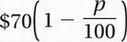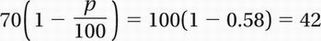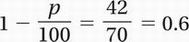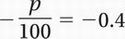# SAT Math Multiple Choice Question 777: Answer and Explanation

### Test Information

Question: 777

12. The original price of an outboard motor was marked down by 30% for a week-long sale. Since the motor was not sold in the first week, it was marked down an additional p percent. If the total markdown from the original price was then 58%, what is the value of p?

• A. 12
• B. 28
• C. 40
• D. 42

Explanation:

C

Algebra (word problems/percent change) HARD

The question makes it clear that we will get the same answer regardless of the original price of the motor. Since we are working with percentages, it is convenient to assume the original price of the motor is \$100.

After a markdown of 30%, the price becomes \$100 - 0.30(\$100) = \$70. If it is marked down an additional p percent, its price becomes. Since this is equivalent to a one-time markdown of 58%, thenDivide by 70:Subtract 1:Multiply by -100:

p = 40